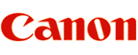PHOTOGRAPHIC GEAR DATE CODE

Most Canon photo gear contains a alpha-numeric date code. For most SLR bodies, this is located in chamber where the film cassette is placed. For flashes, it is located generally just above the focus assist beam projector (some people think this is the serial number of the flash), and lens is usually on the end of the lens mount.

The code contains 2 letter, and 4 numbers.

The first letter is the factor code, the second number is the year code. The next 2 numbers is the month, and the last two numbers is the day of the month.

Year codes:

A='86, B='87, C='88, D='89

E='90, F='91, G='92, H='93, I='94, J='95, K='96, L='97, M='98, N='99

0=2000, P=2001, Q=2002, R=2003, S=2004....

For example OP0910 would be made at factory code "O", in the year 2001, month 09, day 10.